# Integer Type ques of Carbohydrates, Amino Acids, Polymers & Miscellaneous Match the Following, Past JEE Notes | EduRev

## JEE : Integer Type ques of Carbohydrates, Amino Acids, Polymers & Miscellaneous Match the Following, Past JEE Notes | EduRev

The document Integer Type ques of Carbohydrates, Amino Acids, Polymers & Miscellaneous Match the Following, Past JEE Notes | EduRev is a part of the JEE Course Class 12 Chemistry 35 Years JEE Mains &Advance Past yr Paper.
All you need of JEE at this link: JEE

Q. 1. The total number of basic groups in the following form of lysine is           (2010)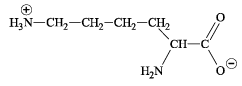Ans. 2

Sol. The basic groups in the given form of lysine is NH2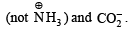Q.2. A decapeptide (Mol. wt. 796) on complete hydrolysis gives glycine (Mol. wt. 75), alanine and phenylalanine. Glycine contributes 47.0% to the total weight of the hydrolysed products. The number of glycine units present in the decapeptide is (2011)

Ans. 6

Sol. Molecular weight of decapeptide = 796 g/mol
Total bonds to be hydrolysed = (10 – 1) = 9 per molecule
Total weight of H2O added = 9 × 18 = 162 g/mol
Total weight of hydrolysis products = 796 + 162 = 958 g
Total weight % of glycine (given) = 47%
Total weight of glycine in product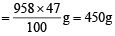Molecular weight of glycine = 75 g/mol

Number of glycine molecules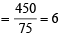Q.3. When the following aldohexose exists in its  D-configuration, the total number of stereoisomers in its pyranose form is : (2012)
CHO — CH2 — CHOH — CHOH — CHOH — CH2OH

Ans. 8

Sol.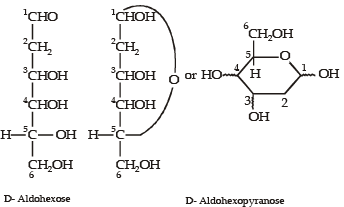Thus, total number of stereoisomers in pyranose form of  D-configuration = 23 = 8

Q.4. The substituents R1 and R2 for nine peptides are listed in the table given below. How many of these peptides are positively charged at pH = 7.0 ?     (2012)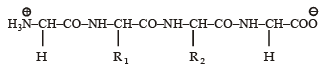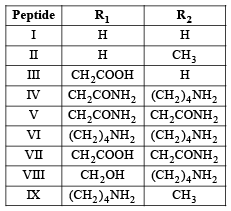Ans. 4

Sol. (4) Peptides with isoelectric point (pI) more than 7, would exist as cation in neutral solution (pH = 7) which means the given polypeptide is of basic nature, so it must contain two or more amino groups. Hence IV, VI, VIII and IX are correct options.

Q.5. A tetrapeptide has —COOH group on alanine. This produces glycine (Gly), valine (Val), phenyl alanine (Phe) and alanine (Ala), on complete hydrolysis. For this tetrapeptide, the number of possible sequences (primary structures) with — NH2 group attached to a chiral center is (JEE Adv. 2013)

Ans.

Sol. (4) According to question C – Terminal must be alanine and N – Terminal do have chiral carbon means it should not be glycine. So possible sequence is :
Val Phe Gly Ala
Val Gly Phe Ala
Phe Val Gly Ala
Phe Gly Val Ala

Q.6. The total number of distinct naturally occurring amino acids obtained by complete acidic hydrolysis of the peptide shown below is (JEE Adv. 2014)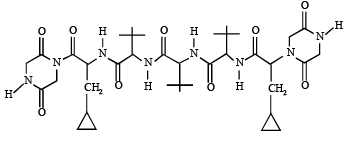Ans. 1

Sol. (1) On hydrolysis, the given peptide gives only one naturally occurring amino acid (glycine).

Offer running on EduRev: Apply code STAYHOME200 to get INR 200 off on our premium plan EduRev Infinity!

69 docs|36 tests

,

,

,

,

,

,

,

,

,

,

,

,

,

,

,

,

,

,

,

,

,

,

,

,

,

,

,

,

,

,

;